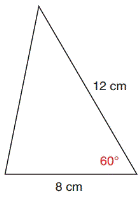Chapter 11.4, Problem 12EElementary Geometry For College St...

7th Edition
Alexander + 2 others
ISBN: 9781337614085

Solutions

Chapter
SectionElementary Geometry For College St...

7th Edition
Alexander + 2 others
ISBN: 9781337614085
Textbook Problem

In Exercises 11 to 14, find the area of each triangle shown. Give the answer to the nearest tenth of a square unit.To determine

To find:

The area of the given triangle

Explanation

Formula:

The area of the triangle is given by,

A=12absinγ unit2.

A=12bcsinα unit2.

A=12acsinβ unit2.

Where a, b, and c are the measures of sides of the triangle, α, β, and γ are the acute angle of the triangle.

Calculation:

Given,

For Δ ABC,

b=8 cm.,

c=12 cm., and

α=60

Since,

Area of the triangle A=12bcsinα unit2

Still sussing out bartleby?

Check out a sample textbook solution.

See a sample solution

The Solution to Your Study Problems

Bartleby provides explanations to thousands of textbook problems written by our experts, many with advanced degrees!

Get Started

Change 36 in. /s to mi/h

Elementary Technical Mathematics

In Exercises 47-52, find and simplify f(a+h)f(a)h(h0) for each function. 50. f(x) = 2x3 x2 + 1

Applied Calculus for the Managerial, Life, and Social Sciences: A Brief Approach

True or False:

Study Guide for Stewart's Multivariable Calculus, 8th